Home | | Computer Applications 11th std | JavaScript Operators and Expressions

# JavaScript Operators and Expressions

An operator combines the values of its operands in some way and evaluates to a new value.

JavaScript Operators and Expressions

An operator combines the values of its operands in some way and evaluates to a new value. Operators are used for JavaScript’s arithmetic expressions, comparison expressions, logical expressions, assignment expressions.An expression is a phrase of JavaScript that a JavaScript interpreter can evaluate to produce a value. The data types are used directly as literals or within variables in combination with simple operators, such as addition, subtraction, and so on, to create an expressions. An expression is a code fragment that can be evaluated to some data type the language supports. An expression is simply one or more variables and/or constants joined by operators. An expression is evaluated and produces a result. The result of all expressions may be either an integer or floating-point value or Boolean value. There are three types of expressions as follows,

Arithmetic expressions

Relational expressions

Logical expressions

## Arithmetic Operators

JavaScript supports all the basic arithmetic operators like addition (+), subtraction (–), multiplication (*), division (/), and modulus (%, also known as the remainder operator).

Table: 14.1 – Arithmetic Operators## Illustration 14.3 Using Arithmetic Operators

<Html>

<Title>Demo Program – To test Arithmetic Operators in JavaScript </Title>

<Body>

<script language="javascript" type="text/javascript">

var value1 = 522, value2=10;

document.write("<br>Data1 : "+value1);

document.write("<br>Data2 : "+value2);

var sum = value1+value2;

var diff = value1-value2;

var prod = value1*value2;

var res = value1/value2;

var rem = value1%value2;

document.write("<br><br>The Sum of Data1 and Data2 : "+sum);

document.write("<br>The Difference of Data1 and Data2 : "+diff);

document.write("<br>The Product of Data1 and Data2 : "+prod);

document.write("<br>The Result after Division of Data1 and Data2 : "+res);

document.write("<br>The Remainder after Division of Data1 and Data2 :"+rem);

</script>

</Body>

</Html>

Output:## Assignment Operator

An assignment operator is the operator used to assign a new value to a variable. Assignment operator can also be used for logical operations such as bitwise logical operations or operations on integral operands and Boolean operands.In JavaScript = is an assignment operator, which is used to assign a value to a variable. Often this operator is used to set a variable to a literal value, for example,

var number1=10;

var number2=number1;

var name=”Computer Science”;

var booleanvar=true;

The assignment operator is used to assign a value to a single variable, but it is possible to perform multiple assignments at once by stringing them together with the = operator. For example, the statement

var m = n = z = 25; // sets all three variables to a value of 25//

The assignment operator can also be used to set a variable to hold the value of an expression. For example,

var x = 102 + 5 - 50; // x set to 57 //

JavaScript supports some shorthand arithmetic operators like +=, -=, *=, /= and %= to evaluate arithmetic calculations.

Table: 14.2 Shorthand Arithmetic operators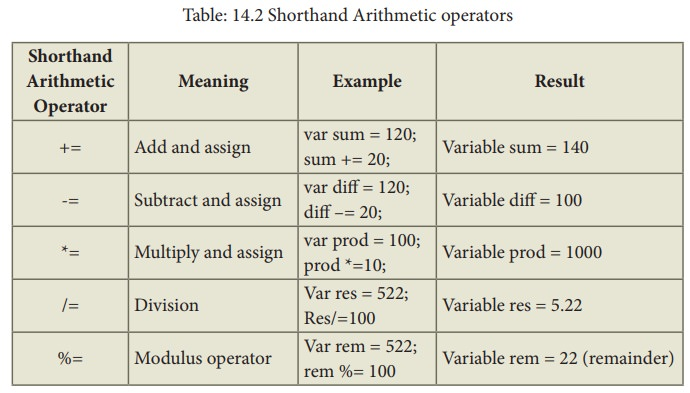## Illustration 14.4 Using Arithmetic Shorthand Operators

<Html>

<Html>

<Title>Demo Program - To test Arithmetic Shorthand Operators in JavaScript

</Title>

<Body>

<script language="javascript" type="text/javascript">

var value1 = 522, value2=10;

document.write("<br>Data1 : "+value1);

document.write("<br>Data2 : "+value2);

var sum = value1; sum+=value2;

var diff = value1; diff-=value2;

var prod = value1; prod*=value2;

var res = value1; res/=value2;

var rem = value1; rem%=value2;

document.write("<br><br>The Sum of Data1 and Data2 Using += : "+sum);

document.write("<br>The Difference of Data1 and Data2 Using -= : "+diff);

document.write("<br>The Product of Data1 and Data2 Using *= : "+prod);

document.write("<br>The Result after Division of Data1 and Data2 using /= : "+res);

document.write("<br>The Remainder after Division of Data1 and Data2 Using %= : "+rem);

</script>

</Body>

</Html>

### Output: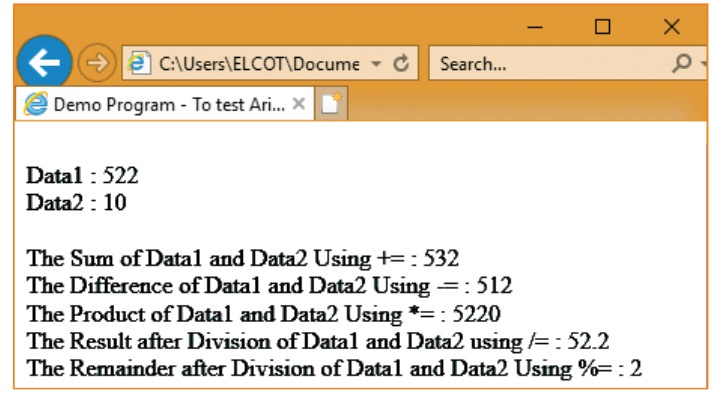Assignment:

Develop JavaScript code for the following:

1.   To find Simple Interest for the given Principle, Number of years and Rate of interest.

2.   To find Compund Interest for the given Principle, Number of years and Rate of interest.

3.   To find difference between Simple Interest and Compound Interst.

## Relational or Comparison Operators:

Relational operators are also called as Comparison operators, they compares two values and the result is true or false. JavaScript provides a rich set of relational operators including == (equal to), != (not equal to), < (less than), > (greater than), <= (less than or equal to), and >= (greater than or equal to). Using a relational operator in an expression causes the expression to evaluate as true if the condition holds or false if otherwise.

Table: 14.3 Relational or Comparison operators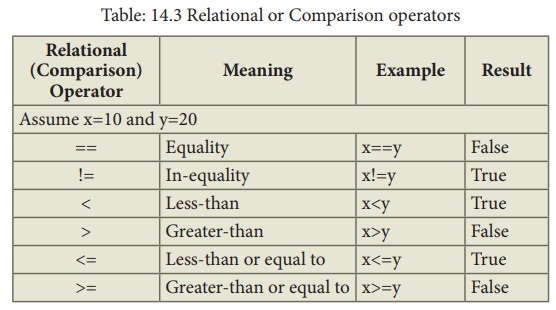## Illustration 14.5 Using Relational Operators

<Html>

<Title>Demo Program - To test Relational(Comparison) Operators in JavaScript </Title>

<Body>

<script language="javascript" type="text/javascript">

var value1 = 522, value2=10;

document.write("<br>Data1 : "+value1);

document.write("<br>Data2 : "+value2);

document.write("<br><br>Whether Data1 = Data2 : "+(value1==value2));

document.write("<br>Whether Data1 < Data2 : "+(value1<value2));

document.write("<br>Whether Data1 > Data2 : "+(value1>value2));

document.write("<br>Whether Data1 <= Data2 : "+(value1<=value2));

document.write("<br>Whether Data1 >= Data2 : "+(value1>=value2));

document.write("<br>Whether Data1 != Data2 : "+(value1!=value2));

</script>

</Body>

</Html>

### Output: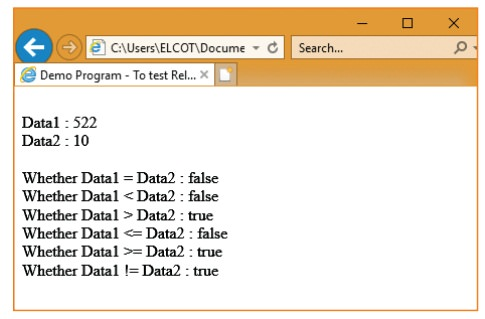## Logical Operators:

Logical operators perform logical (boolean) operations. Logical operators combine or invert boolean values. Once comparisons are made, the logical operators && (AND), || (OR) and ! (NOT) can be used to create more complex conditions.

Table: 14.4 Logical or Boolean operators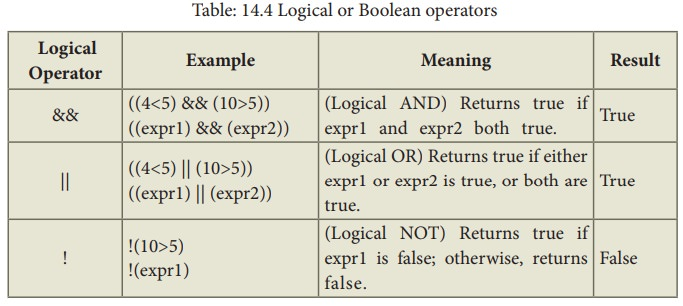### Usage :

Best practice is to use logical operators on boolean operands. However, operands of any type can be combined. The strict rules are as follows:

For && (AND) the result is false if the first operand is false; otherwise, the result is the Boolean value of the second operand.

For || (OR) the result is true if the first operand is true; otherwise, the result is the Boolean value of the second operand.

For ! (NOT) the result is true if the operand is false; otherwise, the result is true.

## Illustration 14.6 Using Logical Operators

<Html>

<Title>Demo Program - To test Logical Operators in JavaScript </Title>

<Body>

<script language="javascript" type="text/javascript">

var value1 = 522, value2=10;

document.write("<br>Data1 : "+value1);

document.write("<br>Data2 : "+value2);

var res1=((value1>100) && (value1>601));

var res2=((value1>100) || (value1>601));

var res3=(!(value1!=value2));

document.write("<br><br>Whether Data1>100 AND Data1>601 : "+res1);

document.write("<br><br>Whether Data1>600 OR Data1>601 : "+res2);

document.write("<br>Whether !Data1 != Data2 : "+res3);

</script>

</Body>

</Html>

### Output:## String Operators:

One of the built-in features of JavaScript is the ability to concatenate strings. The + operator performs addition on numbers but also serves as the concatenation operator for strings. Because string concatenation has precedence over numeric addition, + will be interpreted as string concatenation if any of the operands are strings. + operator which is also called as the string concatenation operator. For example:

## Illustration 14.7 Using + Operator for concatenating String

<Html>

<Title>Demo Program - To Concatenating (+) Operators in JavaScript </Title>

<Body>

<script language="javascript" type="text/javascript">

var String1 = "Java";

var String2 = "Script";

var String3=String1+String2;

document.write("<br>String1 : "+String1);

document.write("<br>String2 : "+String2);

document.write("<br><br>Concatenated String of String1 and String2 : "+String3);

</script>

</Body>

</Html>

Output:## Increment and Decrement Operators:

The ++ operator increments its single operand. The operator converts its operand to a number, adds 1 to that number, and assigns the incremented value back into the variable. The return value of the ++ operator depends on its position relative to the operand. When ++ is used before the operand, where it is known as the pre-increment operator, it increments the operand and evaluates to the incremented value of that operand. When used after the operand, where it is known as the post-increment operator, it increments its operand but evaluates to the un-incremented value of that operand. Consider the difference between these two lines of code:

var m = 1, n = ++m; // m and n are both 2

var m = 1, n = m++; // m is 2, n is 1

The -- operator decrements its single operand. It converts the value of the operand to a number, subtracts 1, and assigns the decremented value back to the operand. Like the ++ operator, the return value of -- depends on its position relative to the operand. When used before the operand, it decrements and returns the decremented value. When used after the operand, it decrements the operand but returns the undecremented value.

var m = 2, n = --m; // m and n are both 1

var m = 2, n = m--; // n is 2, n is 1

## Illustration 14.8 Using ++ and -- Operator – both Prefix and Suffix

<Html>

<Title>Demo Program - ++ and -- Operators in JavaScript </Title>

<Body>

<script language="javascript" type="text/javascript">

var number1 = 150;

var number2 = number1++;

document.write("<br><h4>Post Increment - number++</h4>");

document.write("Number1 = "+number1+" Number2 = "+number2);

document.write("<br><h4>Pre Increment - ++number</h4>"); var number1 = 150;

var number2 = ++number1;

document.write("Number1 = "+number1+" Number2 = "+number2);

var number1 = 150;

var number2 = number1--;

document.write("<br><h4>Post Decrement - number--</h4>");

document.write("Number1 = "+number1+" Number2 = "+number2);

document.write("<br><h4>Pre Decrement - --number</h4>");

var number1 = 150;

var number2 = --number1;

document.write("Number1 = "+number1+" Number2 = "+number2);

</script>

</Body>

</Html>

### Output:## Unary + and - Operator:

+ has no effect on numbers but causes non-numbers to be converted into numbers

– Negation (changes the sign of the number or converts the expression to a number and then changes its sign)

## typeof Operator:

The typeof operator is used to get the data type (returns a string) of its operand. The operand can be either a literal or a data structure such as a variable, a function, or an object. The operator returns the data type.

### Syntax

typeof operand

or

typeof(operand)

typeof returns: boolean, function, number, string, and undefined. The following table summarizes possible values returned by the typeof operator.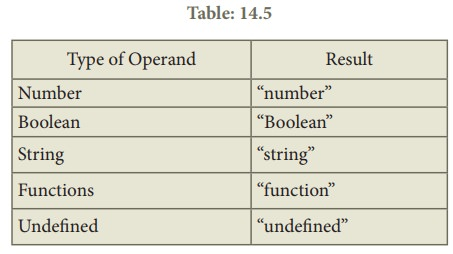## Illustration 14.9 typeof operator

<Html>

<Title>Demo Program - To test typeof Operator in JavaScript </Title>

<Body>

<script language="javascript" type="text/javascript">

var value1 = 522, value2="JavaSript";

value3=true;

document.write("<br>Value1 ="+value1+" and its data Type is : "+typeof(value1));

document.write ("<br>Value2 ="+value2+" and its data Type is : "+typeof(value2));

document.write ("<br>Value3 ="+value3+" and its data Type is : "+typeof(value3));

</script>

</Body>

</Html>

### Output:## Conditional Operator (?:)

The ?: is the conditional operator in JavaScript, which requires three operands, hence it is called the ternary operator. The syntax is

var variablename=(condition) ? value1 : value2;

In the syntax condition may be relational expression or logical expression. First condition will be evaluated, if the condition returns true then the value of the left side of the colon is assigned to the variable otherwise the value of the right side of the colon will be assigned the variable. For example,

var result=(10>15) ?100 :150;

In the above example, since the condition returns false the value 150 will be assigned to result.

## Illustration 14.10 Condtional Operator

<Html>

<Title>Demo Program - To test Conditional Operator in JavaScript </Title>

<Body>

<script language="javascript" type="text/javascript">

var value1 = 522, value2=150, value3;

value3=(value1<value2) ? value1: value2;

document.write("<br>The Value of Data1 = "+value3);

</script>

</Body>

</Html>

### Output:Study Material, Lecturing Notes, Assignment, Reference, Wiki description explanation, brief detail
11th Computer Applications : Chapter 14 : Introduction to JavaScript : JavaScript Operators and Expressions |